# Euler-MacLaurin formula

(Redirected from Euler–MacLaurin formula)
Jump to: navigation, search

A summation formula that connects the partial sums of a series with the integral and derivatives of its general term: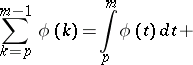where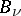are the Bernoulli numbers and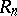is the remainder. Using the Bernoulli polynomials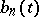,, the remainder can be rewritten in the form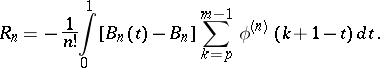For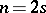the remaindercan be expressed by means of the Bernoulli numbers: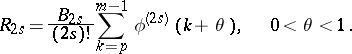If the derivativesand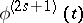have the same sign and do not change sign on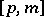, then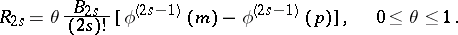If, furthermore,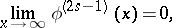then the Euler–MacLaurin formula becomes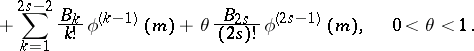This version is used, for example, to derive the Stirling formula, in which caseandis the Euler constant. The formula has also been generalized to multiple sums.

The Euler–MacLaurin formula finds application in the approximate calculation of definite integrals, the study of convergence of series, the computation of sums, and the expansion of functions in Taylor series. For example, for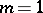,,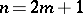, and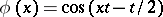, it yields the expression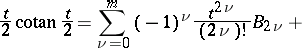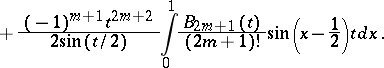The Euler–MacLaurin formula plays an important role in the study of asymptotic expansions, number-theoretic estimates and finite-difference calculus.

Sometimes the Euler–MacLaurin formula is applied in the form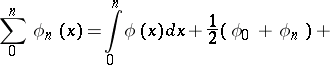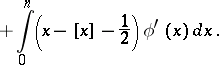The formula was first obtained by L. Euler  aswhereis the sum of the first terms of the series with general term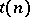,for, and the coefficients are determined from the recurrence relations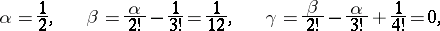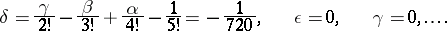The formula was later discovered independently by C. MacLaurin .